﻿

### A viscoelastic fluid flow through mixing grids

#### Abstract

We study the asymtotic behaviour of a viscoelastic fluid in a porous medium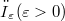$ω_\varepsilon (\varepsilon>0)$ obtained by removing from an open set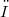$ω$ small obstacles$(T\varepsilonv)_{1≤{v}≤{n(\varepsilon)}}$ of size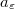$a_\varepsilon$ periodically distributed on a hyperplane H which intersects$ω$. We establish that the fluid behave differently depending on whether the size$a_\varepsilon$ is greater than or smaller than a critical size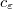$c_\varepsilon$. If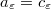$a_\varepsilon = c_\varepsilon$ , a convolution term appears in the limit problem. This corresponds to a long memory effect. If$a_\varepsilon$ is smaller than$c_\varepsilon$, the fluid behaves as if there where no obstacles. If$a_\varepsilon$ is greater than$c_\varepsilon$ or is of the order of the period, the fluid adheres on the hyperplane H which plays a thin solid plate role and the fluid behaves separately on each side of this plate.

DOI Code: 10.1285/i15900932v19n2p153

Full Text: PDF